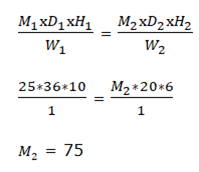# NIACL AO Prelims – Quantitative Aptitude Questions 2021 Day- 01

Dear Aspirants, Our IBPS Guide team is providing new series of Quantitative Aptitude Questions for NIACL AO Prelims 2021 so the aspirants can practice it on a daily basis. These questions are framed by our skilled experts after understanding your needs thoroughly. Aspirants can practice these new series questions daily to familiarize with the exact exam pattern and make your preparation effective.

### Start Quiz

Mixture and allegation

1) Vessel A contains 90 liters milk which is 60% of the quantity of vessel A and rest of quantity is water. If x liters of milk and (x + 10) liters of water is added to vessel A, then the ratio of the milk and water becomes 6:5. If vessel B contains (x + 30) liters mixture of milk and water in the ratio of 3:2, then find the quantity of milk in vessel B?

A.45 liters

B.30 liters

C.36 liters

D.48 liters

E.42 liters

Time and work

2) If 25 men can do a piece of work in 36 days working 10 hours a day, then how many men are required to complete the work working 6 hours a day in 20 days?

A.85

B.80

C.75

D.95

E.70

Ratio and proportion

3) The ratio of the total number of CSE students and ECE students is 5:4 and the ratio of number of boys in CSE department is 40% more than the number of boys in ECE departments. If the number of girls in CSE department is 170, then find the number of boys in ECE?

A.200

B.150

C.100

D.250

E.Cannot be determined

Partnership

4) A, B and C started the business with the investment in the ratio of 4:3:5 and the investment period of A, B and C is 10 months, 12 months and 8 months respectively. What is the ratio of profit of A, B and C?

A.5:4:6

B.3:2:3

C.10:9:10

D.6:5:6

E.None of these

Profit and loss

5) The marked price of the AC is 20% more than its cost price and the selling price of the AC is 15% more than its cost price. If the shopkeeper offers a discount of Rs.500 on marked price of AC, then find the selling price of AC?

A.Rs.12000

B.Rs.11800

C.Rs.12400

D.Rs.11500

E.None of these

Directions (06-10): Following question contains two equations as I and II. You have to solve both equations and determine the relationship between them and give answer as,

6) I) 3x + 4y = 45

II)2x + 3y = 32

A.x > y

B.x ≥ y

C.x = y or relationship can’t be determined.

D.x < y

E.x ≤ y

7) I)
x2 – x – 600 = 0

II)y2+ 27y + 72 = 0

A.x > y

B.x ≥ y

C.x = y or relationship can’t be determined.

D.x < y

E.x ≤ y

8) I)
2x2 + 26x + 84 = 0

II)y2+ 15y + 56 = 0

A.x > y

B.x ≥ y

C.x = y or relationship can’t be determined.

D.x < y

E.x ≤ y

9) I)
x2 + 29x + 210 = 0

II)y2+ 2y – 168 = 0

A.x > y

B.x ≥ y

C.x = y or relationship can’t be determined.

D.x < y

E.x ≤ y

10) I)
x2 + 178 = 502

II)y2= 361

A.x > y

B.x ≥ y

C.x = y or relationship can’t be determined.

D.x < y

E.x ≤ y

### Try NIACL AO Online Practice Mock Test

Quantity of water in vessel A = 40/60 * 90 = 60 liters

(90 + x)/(60 + x + 10) = 6/5

450 + 5x = 420 + 6x

x = 30 liters

Quantity of vessel B = 30 + 30 = 60 liters

Milk in vessel B = 3/5 * 60 = 36 litersTotal number of CSE students = 5x

Total number of ECE students = 4x

Number of boys in ECE students = 5y

Number of boys in CSE students = 5y * 140/100 = 7y

5x – 7y = 170

Required ratio = 4x * 10:3x * 12:5x * 8

= 40:36:40

= 10:9:10

CP of AC = 5x

MP of AC = 5x * 120/100 = 6x

SP of AC = 5x * 115/100 = 5.75x

6x – 500 = 5.75x

x = 2000

SP of AC = 5.75 * 2000 = Rs.11500

3x + 4y = 45 —–(1)

2x + 3y = 32 —–(2)

From (1) and (2)

y = 6

3x = 45 – 24

x = 7

x > y

x2 – x – 600 = 0

x2 – 25x + 24x – 600 = 0

x(x – 25) + 24(x – 25) = 0

(x + 24)(x – 25) = 0

x = -24, 25

y2 + 27y + 72 = 0

y2 + 24y + 3y + 72 = 0

y(y + 24) + 3(y + 24) = 0

(y + 3)(y + 24) = 0

y = -3, -24

Relationship between x and y cannot be established.

2x2 + 26x + 84 = 0

2x2 + 14x + 12x + 84 = 0

2x(x + 7) + 12(x + 7) = 0

(2x + 12)(x + 7) = 0

x = -6, -7

y2 + 15y + 56 = 0

y2 + 8y + 7y + 56 = 0

y(y + 8) + 7(y + 8) = 0

(y + 7)(y + 8) = 0

y = -7, -8

x ≥ y

x2 + 29x + 210 = 0

x2 + 14x + 15x + 210 = 0

x(x + 14) + 15(x + 14) = 0

(x + 15)(x + 14) = 0

x = -15, -14

y2 + 2y – 168 = 0

y2 – 12y + 14y – 168 = 0

y(y – 12) + 14(y – 12) = 0

(y + 14)(y – 12) = 0

y = -14, 12

x ≤ y

x2 + 178 = 502

x2 = 324

x = 18, -18

y2 = 361

y = 19, -19

Relationship between x and y cannot be established.